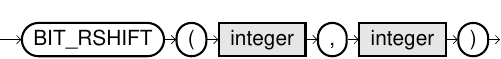# BIT_RSHIFT

## Purpose

This function shifts the bits of a number by the specified number to the right.

## Syntax

bit_rshift::=## Usage Notes

• Bit functions are limited to 64 bits, which is positive numbers between 0 and 18446744073709551615.
• The second parameter can be between 0 and 63.
• The result data type is DECIMAL(20,0).

## Example

``SELECT BIT_RSHIFT(1024,10);``